# Activity: Find an Approximate Value For PiYou will need: A piece of card A compass and pencil A protractor A ruler A pair of scissors Glue and paper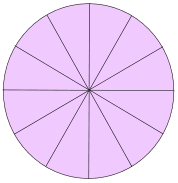## Step 1

Draw a circle on your card. The exact size doesn't matter, but let's use a radius of 5 cm (centimeters).

Use your protractor to divide the circle up into twelve equal sectors.

What is the angle for each sector? That's easy – just divide 360° (one complete turn) by 12:

360° / 12 = 30°

So each of the angles must be 30°

## Step 2

Divide just one of the sectors into two equal parts – that's 15° for each sector.

You now have thirteen sectors – number them 1 to 13:## Step 3

Cut out the thirteen sectors using the scissors: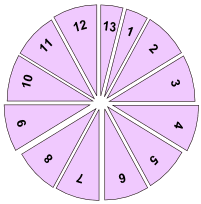## Step4

Rearrange the 13 sectors like this (you can glue them onto a piece of paper):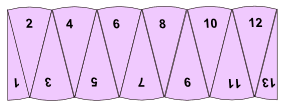Now that shape resembles a rectangle: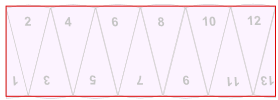## Step 5

What are the (approximate) height and width of the rectangle?

Its height is the circle's radius: just look at sectors 1 and 13 above. When they are in the circle they are "radius" high.

Its width (actually one "bumpy" edge), is half of the curved parts around the circle ... in other words it is about half the circumference of the original circle. We know that:

Circumference = 2 × π × radius

And so the width is:

Half the Circumference = π × radius

And so we have (approximately):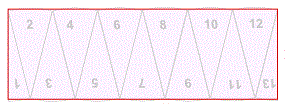radius π × radius

With a radius of 5 cm, the rectangle should be:

• 5 cm high

## Step 6

Measure the actual length of your "rectangle" as accurately as you can using your ruler.

Divide by the radius (5 cm) to get an approximation for π

 "Rectangle" Width Divide by 5 cm ≈ π